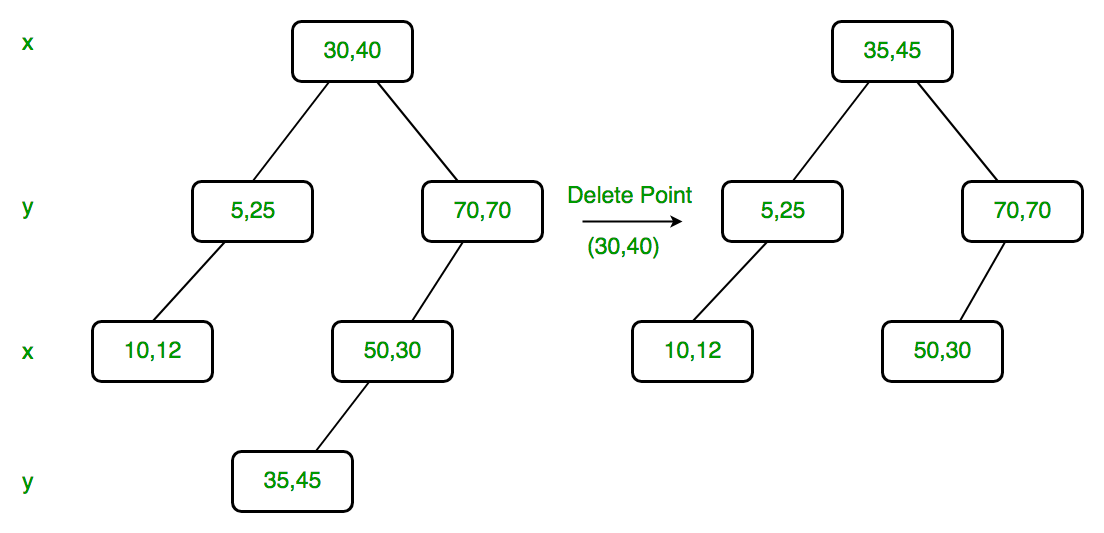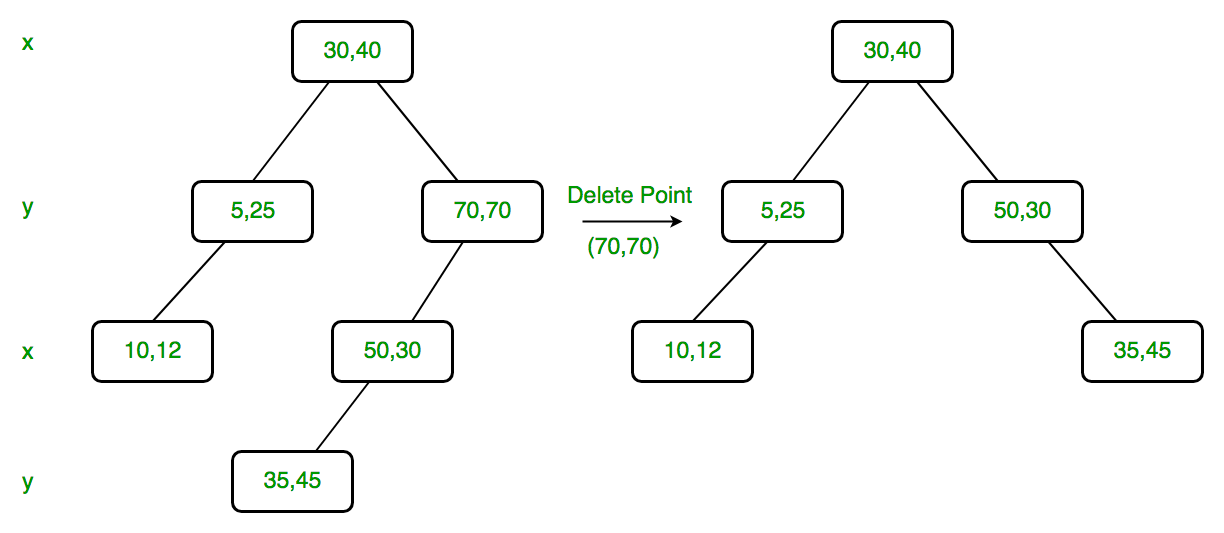# K Dimensional Tree | Set 3 (Delete)

• Difficulty Level : Hard
• Last Updated : 17 Sep, 2021

We strongly recommend to refer below posts as a prerequisite of this.
K Dimensional Tree | Set 1 (Search and Insert)
K Dimensional Tree | Set 2 (Find Minimum)
In this post delete is discussed. The operation is to delete a given point from K D Tree.

Like Binary Search Tree Delete, we recursively traverse down and search for the point to be deleted. Below are steps are followed for every node visited.

1) If current node contains the point to be deleted

1. If node to be deleted is a leaf node, simply delete it (Same as BST Delete)
2. If node to be deleted has right child as not NULL (Different from BST)
1. Find minimum of current node’s dimension in right subtree.
2. Replace the node with above found minimum and recursively delete minimum in right subtree.
3. Else If node to be deleted has left child as not NULL (Different from BST)
1. Find minimum of current node’s dimension in left subtree.
2. Replace the node with above found minimum and recursively delete minimum in left subtree.
3. Make new left subtree as right child of current node.

2) If current doesn’t contain the point to be deleted

1. If node to be deleted is smaller than current node on current dimension, recur for left subtree.
2. Else recur for right subtree.

Why 1.b and 1.c are different from BST?
In BST delete, if a node’s left child is empty and right is not empty, we replace the node with right child. In K D Tree, doing this would violate the KD tree property as dimension of right child of node is different from node’s dimension. For example, if node divides point by x axis values. then its children divide by y axis, so we can’t simply replace node with right child. Same is true for the case when right child is not empty and left child is empty.

Why 1.c doesn’t find max in left subtree and recur for max like 1.b?
Doing this violates the property that all equal values are in right subtree. For example, if we delete (!0, 10) in below subtree and replace if with

```Wrong Way (Equal key in left subtree after deletion)
(5, 6)                             (4, 10)
/              Delete(5, 6)         /
(4, 10)            ------------>     (4, 20)
\
(4, 20)

Right way (Equal key in right subtree after deletion)
(5, 6)                          (4, 10)
/              Delete(5, 6)           \
(4, 10)            ------------>         (4, 20)
\
(4, 20) ```

Example of Delete:
Delete (30, 40): Since right child is not NULL and dimension of node is x, we find the node with minimum x value in right child. The node is (35, 45), we replace (30, 40) with (35, 45) and delete (30, 40).Delete (70, 70): Dimension of node is y. Since right child is NULL, we find the node with minimum y value in left child. The node is (50, 30), we replace (70, 70) with (50, 30) and recursively delete (50, 30) in left subtree. Finally we make the modified left subtree as right subtree of (50, 30).Below is C++ implementation of K D Tree delete.

## C++

 `// A C++ program to demonstrate delete in K D tree``#include``using` `namespace` `std;`` ` `const` `int` `k = 2;`` ` `// A structure to represent node of kd tree``struct` `Node``{``    ``int` `point[k]; ``// To store k dimensional point``    ``Node *left, *right;``};`` ` `// A method to create a node of K D tree``struct` `Node* newNode(``int` `arr[])``{``    ``struct` `Node* temp = ``new` `Node;`` ` `    ``for` `(``int` `i=0; ipoint[i] = arr[i];`` ` `    ``temp->left = temp->right = NULL;``    ``return` `temp;``}`` ` `// Inserts a new node and returns root of modified tree``// The parameter depth is used to decide axis of comparison``Node *insertRec(Node *root, ``int` `point[], unsigned depth)``{``    ``// Tree is empty?``    ``if` `(root == NULL)``        ``return` `newNode(point);`` ` `    ``// Calculate current dimension (cd) of comparison``    ``unsigned cd = depth % k;`` ` `    ``// Compare the new point with root on current dimension 'cd'``    ``// and decide the left or right subtree``    ``if` `(point[cd] < (root->point[cd]))``        ``root->left = insertRec(root->left, point, depth + 1);``    ``else``        ``root->right = insertRec(root->right, point, depth + 1);`` ` `    ``return` `root;``}`` ` `// Function to insert a new point with given point in``// KD Tree and return new root. It mainly uses above recursive``// function "insertRec()"``Node* insert(Node *root, ``int` `point[])``{``    ``return` `insertRec(root, point, 0);``}`` ` `// A utility function to find minimum of three integers``Node *minNode(Node *x, Node *y, Node *z, ``int` `d)``{``    ``Node *res = x;``    ``if` `(y != NULL && y->point[d] < res->point[d])``       ``res = y;``    ``if` `(z != NULL && z->point[d] < res->point[d])``       ``res = z;``    ``return` `res;``}`` ` `// Recursively finds minimum of d'th dimension in KD tree``// The parameter depth is used to determine current axis.``Node *findMinRec(Node* root, ``int` `d, unsigned depth)``{``    ``// Base cases``    ``if` `(root == NULL)``        ``return` `NULL;`` ` `    ``// Current dimension is computed using current depth and total``    ``// dimensions (k)``    ``unsigned cd = depth % k;`` ` `    ``// Compare point with root with respect to cd (Current dimension)``    ``if` `(cd == d)``    ``{``        ``if` `(root->left == NULL)``            ``return` `root;``        ``return` `findMinRec(root->left, d, depth+1);``    ``}`` ` `    ``// If current dimension is different then minimum can be anywhere``    ``// in this subtree``    ``return` `minNode(root,``               ``findMinRec(root->left, d, depth+1),``               ``findMinRec(root->right, d, depth+1), d);``}`` ` `// A wrapper over findMinRec(). Returns minimum of d'th dimension``Node *findMin(Node* root, ``int` `d)``{``    ``// Pass current level or depth as 0``    ``return` `findMinRec(root, d, 0);``}`` ` `// A utility method to determine if two Points are same``// in K Dimensional space``bool` `arePointsSame(``int` `point1[], ``int` `point2[])``{``    ``// Compare individual pointinate values``    ``for` `(``int` `i = 0; i < k; ++i)``        ``if` `(point1[i] != point2[i])``            ``return` `false``;`` ` `    ``return` `true``;``}`` ` `// Copies point p2 to p1``void` `copyPoint(``int` `p1[], ``int` `p2[])``{``   ``for` `(``int` `i=0; ipoint, point))``    ``{``        ``// 2.b) If right child is not NULL``        ``if` `(root->right != NULL)``        ``{``            ``// Find minimum of root's dimension in right subtree``            ``Node *min = findMin(root->right, cd);`` ` `            ``// Copy the minimum to root``            ``copyPoint(root->point, min->point);`` ` `            ``// Recursively delete the minimum``            ``root->right = deleteNodeRec(root->right, min->point, depth+1);``        ``}``        ``else` `if` `(root->left != NULL) ``// same as above``        ``{``            ``Node *min = findMin(root->left, cd);``            ``copyPoint(root->point, min->point);``            ``root->right = deleteNodeRec(root->left, min->point, depth+1);``        ``}``        ``else` `// If node to be deleted is leaf node``        ``{``            ``delete` `root;``            ``return` `NULL;``        ``}``        ``return` `root;``    ``}`` ` `    ``// 2) If current node doesn't contain point, search downward``    ``if` `(point[cd] < root->point[cd])``        ``root->left = deleteNodeRec(root->left, point, depth+1);``    ``else``        ``root->right = deleteNodeRec(root->right, point, depth+1);``    ``return` `root;``}`` ` `// Function to delete a given point from K D Tree with 'root'`` ``Node* deleteNode(Node *root, ``int` `point[])``{``   ``// Pass depth as 0``   ``return` `deleteNodeRec(root, point, 0);``}`` ` `// Driver program to test above functions``int` `main()``{``    ``struct` `Node *root = NULL;``    ``int` `points[][k] = {{30, 40}, {5, 25}, {70, 70},``                      ``{10, 12}, {50, 30}, {35, 45}};`` ` `    ``int` `n = ``sizeof``(points)/``sizeof``(points);`` ` `    ``for` `(``int` `i=0; ipoint << ``", "` `<< root->point << endl;`` ` `    ``return` `0;``}`

Output:

```Root after deletion of (30, 40)
35, 45```

Source:
https://www.cs.umd.edu/class/spring2008/cmsc420/L19.kd-trees.pdf